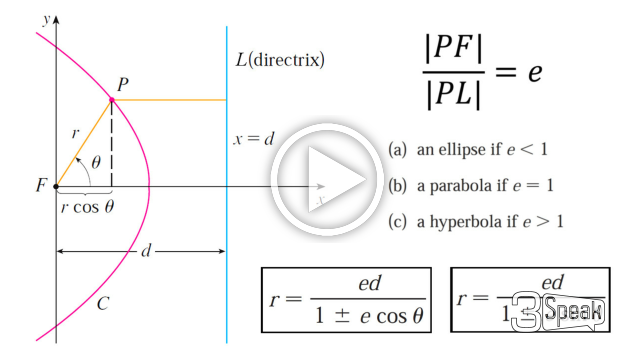# Conics in Polar Coordinates: Unified Theorem for Conic Sections

in Threespeak15 days agoIn this video I go over further into Conic Sections and this time go over a more “Unified” theorem for conics that has the benefit of being written down as a simple formula in Polar Coordinates. From my earlier videos on Conic Sections, I went over the conventional theorems for defining Parabolas, Ellipses, and Hyperbolas; which were defined in Cartesian or Rectangular Coordinates. But the issue with the conventional theorems is that Parabolas were defined with a Focus and a Directrix, while Ellipses and Hyperbolas were defined as having two Foci. A more unified approach is to use just one theorem that encompasses all of the conic sections. I go over a summary of such a theorem and which just involves a Focus and a Directrix.

The Theorem states that the ratio of the distance from a point to the Focus divided by the distance from the point to the Directrix is equal to e and is called the Eccentricity. When e = 1 the conic is a parabola; when e is greater than 1 the conic is a Hyperbola; and when e is less than 1 the conic is an ellipse. In this video I go just over a summary of this theorem but in later videos I will go over the proof to show that this does in fact represent conic sections as typically defined in my earlier videos.

I also show that this more Unified Theorem has the benefit that when the focus is placed at the origin (or Pole), then this theorem can be written in a short and simple equation in Polar Coordinates. Depending on where the Directrix is, the formula will be slightly modified; which I will explain in more detail in a later video so stay tuned for that as well! This is a great video in both revisiting conic sections but also in explaining how sometimes we can produce more efficient theorems when changing up the coordinate system, so make sure to watch this video!

View video notes on the Hive blockchain: https://peakd.com/steemstem/@mes/conics-in-polar-coordinates-unified-theorem-for-conic-sections

Related Videos:

Conic Sections: Hyperbola: Definition and Formula: https://youtu.be/UBIHovXNV9U
Conic Sections: Ellipses: Definition and Derivation of Formula (Including Circles): https://youtu.be/9dETsJ2tz_M
Conic Sections: Parabolas: Definition and Formula: https://youtu.be/kCJjXuuIqbE
Polar Coordinates: Cartesian Connection: https://youtu.be/HcaTYrpmGaU
Polar Coordinates: Infinite Representations: https://youtu.be/QJYbnO7NzCk

SUBSCRIBE via EMAIL: https://mes.fm/subscribe

DONATE! ʕ •ᴥ•ʔ https://mes.fm/donate

Like, Subscribe, Favorite, and Comment Below!

Official Website: https://MES.fm
Hive: https://peakd.com/@mes
Gab: https://gab.ai/matheasysolutions
Minds: https://minds.com/matheasysolutions
Pinterest: https://pinterest.com/MathEasySolns
Instagram: https://instagram.com/MathEasySolutions
Email me: [email protected]

Try our Free Calculators: https://mes.fm/calculators

BMI Calculator: https://bmicalculator.mes.fm
Mortgage Calculator: https://mortgagecalculator.mes.fm
Percentage Calculator: https://percentagecalculator.mes.fm

Try our Free Online Tools: https://mes.fm/tools

iPhone and Android Apps: https://mes.fm/mobile-apps

▶️ 3Speak

Sort:

I don't always go over a Unified Theorem for Conics but when I do it's usually to be able to write the theorem in a simple Polar Equation ;)

View video notes on the Hive blockchain: https://peakd.com/steemstem/@mes/conics-in-polar-coordinates-unified-theorem-for-conic-sections